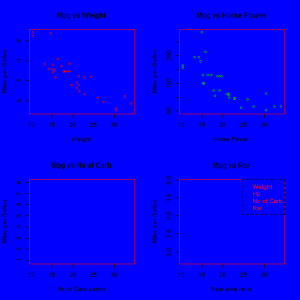Want to share your content on R-bloggers? click here if you have a blog, or here if you don't.In the exercises below we practice how to personalize graphics parameters, how to produce different plots at the same time and how to save a plot in a file. We will use commands such as `par` and `jpeg`. We will use the mtcars dataset, provided by R Cran (we can upload the dataset by typing ` mtcars ` and then attach our dataset by ` attach(mtcars)`). A description of the data is available here.

This set is the third set of exercises is a series on data visualization. Click here for the other sets in the series. Answers to the exercises are available here.

If you obtained a different (correct) answer than those listed on the solutions page, please feel free to post your answer as a comment on that page.

Exercise 1
We are bored to see classic plots and modify every time colors and other details. So, we want to set by default a blue background colour and red dots/lines. What command should we use?

a.`par(col=2,lty=2,bg=4);plot(mpg,wt)`
b.`plot(mpg,wt,col=2,bg=4)`

Exercise 2

Suppose we want to see more than one plot at time. What command do we have to use to see 2 plots by row?

a.`par(mfrow=c(1,2));plot(mpg,wt);plot(mpg,hp)`
b.`par(mfrow=c(2,1));plot(mpg,wt);plot(mpg,hp)`

Exercise 3

Now we have a general vision to how set and personalize graphics tools in R. In this exercise we have to produce 4 different plots at the same time, 2 by rows and 2 by columns. In addition we have to add some details as: dots colour, labels, titles and an overall legend.

Exercise 4

After exercise 3, we want to turn back to default settings of our machine. How we can reset our settings with one command?

a.`dev.off=0`
b.`dev.off()`

Exercise 5

Plots in exercise 3 seemed to be too much close each other. The solution is set margins of our background plot panel. How can we accomplish this?

a.`par(mar=c(4, 4, 4, 4))`
b.`par(tck=c(1, 2, 2, 1))`

Exercise 6

RStudio gives us the opportunity to personalize theme and panels. But we are lazy and we want to set only console and Source panel gave them all available space. How we can plot in other window?

a.`windows();plot(mpg,cyl)`
b.`dev.in();plot(mpg,cyl)`

Exercise 7

Now, we know well how to set graphic parameters and plot details. So we don’t want to waste our time seeing the plot in a panel, and instead we want to save directly to a jpeg file. What do we have to do?

a.```ex7<-plot(mpg,cyl);jpeg(filename = "Rplot.jpg", + width = 480, height = 480, units = "px", pointsize = 12, + quality = 75, + bg = "white", res = NA, family = "", restoreConsole = TRUE, + type = c("windows", "cairo"), ex7)```

b.```ex7<-plot(mpg,cyl);save.plot(filename = "Rplot.jpg", + width = 480, height = 480, units = "px", pointsize = 12, + quality = 75, + bg = "white", res = NA, family = "", restoreConsole = TRUE, + type = c("windows", "cairo"), ex7)```

Exercise 8

Finally we want to produce a summary of what we have learned from these exercises. So, prepare one plot with 4 variables (see previous exercises), personalize them and save them into a jpeg file.